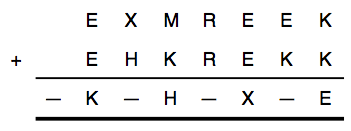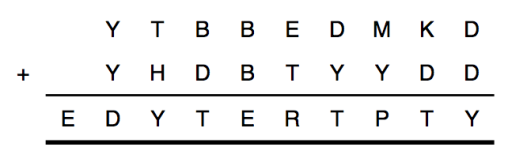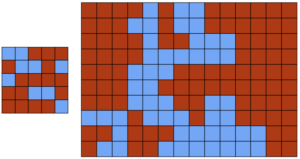## A cube of primes

This week’s Riddler Classic is a question about prime numbers.

Consider a cube, which has eight vertices, or corners. Suppose I assign a prime number to each vertex. A “face sum” is the value I get when I add up all four prime numbers on one of the six faces.

Can you find eight distinct primes and arrange them on a cube so that the six face sums are all equal?

Extra credit: Can you find another set of eight distinct primes that can similarly be arranged on the vertices of a cube? How many more can you find?

Extra Extra credit: Same puzzle for the other four platonic solids.

Here is my solution:
[Show Solution]

## Smudged secret messages

This Riddler is a twist on a classic problem: decoding equations! Here is the paraphrased problem:

The goal is to decode two equations. In each of them, every different letter stands for a different digit. But there is a minor problem in both equations. In the first equation, letters accidentally were smudged and are now unreadable. (These are represented with dashes below.) But we know that all 10 digits, 0 through 9, appear in the equation.What digits belong to what letters, and what are the dashes? In the second equation, one of the letters in the equation is wrong. But we don’t know which one. Which is it?Here is my detailed solution:
[Show Solution]

If you’re just interested in the answers, here they are:
[Show Solution]

## Ostomachion coloring

The following problems appeared in The Riddler. And it features an interesting combination of an ancient game with the four-color theorem.

The famous four-color theorem states, essentially, that you can color in the regions of any map using at most four colors in such a way that no neighboring regions share a color. A computer-based proof of the theorem was offered in 1976.

Some 2,200 years earlier, the legendary Greek mathematician Archimedes described something called an Ostomachion (shown below). It’s a group of pieces, similar to tangrams, that divides a 12-by-12 square into 14 regions. The object is to rearrange the pieces into interesting shapes, such as a Tyrannosaurus rex. It’s often called the oldest known mathematical puzzle.

Your challenge today: Color in the regions of the Ostomachion square with four colors such that each color shades an equal area. (That is, each color needs to shade 36 square units.) The coloring must also satisfy the constraint that no adjacent regions are the same color.

Extra credit: How many solutions to this challenge are there?Here are the details of how I solved the problem:
[Show Solution]

To jump straight to the solution (and pretty pictures!) see below.
[Show Solution]

## Squaring the square

This Riddler puzzle is about tiling a square using smaller squares.

You are handed a piece of paper containing the 13-by-13 square shown below, and you must divide it into some smaller square pieces. If you are only allowed to cut along the lines, what is the smallest number of squares you can divide this larger square into? (You could, for example, divide it into one 12-by-12 square and 25 one-by-one squares for a total of 26 squares, but you can do much better.)Here is how I solved the problem:
[Show Solution]

And here is the tl;dr, just the solutions!
[Show Solution]

## Timing a stoplight just right

This Riddler is about how to perfectly time a stoplight, something we’ve all had to deal with!

You are driving your car on a perfectly flat, straight road. You are the only one on the road and you can see anything ahead of you perfectly. At time t=0, you are at Point A, cruising along at a speed of 100 kilometers per hour, which is the speed limit for the whole road. You want to reach Point C, exactly 4 kilometers ahead, in the shortest time possible. But, at Point B, 2 kilometers ahead of you, there is a traffic light.

At time t=0, the light is green, but you don’t know how long it has been green. You do know that at the beginning of each second, there is a 1 percent chance that the light will turn yellow. Once it turns yellow, it remains yellow for 5 seconds and then turns red for 20 seconds. Your car can accelerate or decelerate at a maximum rate of 2 meters per second-squared. You must always drive at or below the speed limit. You can pass through the intersection when the traffic light is yellow, but not when it is red.

What is the best strategy to reach your destination as soon as possible?

Here is my solution:
[Show Solution]

## Rig the election with math!

This Riddler problem is about gerrymandering. How to redraw borders to sway a vote one way or another.

Below is a rough approximation of Colorado’s voter preferences, based on county-level results from the 2012 presidential election, in a 14-by-10 grid. Colorado has seven districts, so each would have 20 voters in this model. In each district, the party with the most votes will win. The districts must be non-overlapping and contiguous (that is, each square in a district must share an edge with at least one other square in the district). What is the most districts that the Red Party could win? What about the Blue Party? (Assume ties within a district are considered wins for the party of your choice.)

Two boards are provided, a test 5×5 grid and the larger 14×10 grid:Here is a first solution, using some simple logic and intuition:
[Show Solution]

And here is a computational approach, using integer programming:
[Show Solution]

## Non-intersecting chessboard paths

This Riddler classic puzzle is about finding non-intersecting paths on a chessboard:

First, how long is the longest path a knight can travel on a standard 8-by-8 board without letting the path intersect itself?

Second, there are unorthodox chess pieces that don’t exist in the standard game, which are known as fairy chess pieces. What are the longest nonintersecting paths that can be taken by the camel (which moves like a knight, except 3 squares by 1 square), the zebra (3 by 2), and the giraffe (4 by 1)?

This is a very challenging problem, and there doesn’t appear to be any way to solve it via some clever observation or simplification. Of course, we can try to come up with ever longer tours by hand, but we’ll never know for sure that we have found the longest one.

Much like the recent Pokemon Go problem, we must resort to computational means to obtain a solution. In this case, however, the problem is “small enough” that we can find exact solutions!

Here are some optimal tours:
[Show Solution]

If you’re interested in the details of how I found my solutions, read on:
[Show Solution]

## Pokémon Go Efficiency

This Riddler puzzle is about a topic near and dear to many hearts: Pokémon!

Your neighborhood park is full of Pokéstops — places where you can restock on Pokéballs to, yes, catch more Pokémon! You are at one of them right now and want to visit them all. The Pokéstops are located at points whose (x, y) coordinates are integers on a fixed coordinate system in the park.

For any given pair of Pokéstops in your park, it is possible to walk from one to the other along a path that always goes from one Pokéstop to another Pokéstop adjacent to it. (Two Pokéstops are considered adjacent if they are at points that are exactly 1 unit apart. For example, Pokéstops at (3, 4) and (4, 4) would be considered adjacent.)

You’re an ambitious and efficient Pokémon trainer, who is also a bit of a homebody: You wish to visit each Pokéstop and return to where you started, while traveling the shortest possible total distance. In this open park, it is possible to walk in a straight line from any point to any other point — you’re not confined to the coordinate system’s grid. It turns out that this is a really hard problem, so you seek an approximate solution.

If there are N Pokéstops in total, find the upper and lower bounds on the total length of the optimal walk. (Your objective is to find bounds whose ratio is as close to 1 as possible.)

Advanced extra credit: For solvers who prefer a numerical question with this theme, suppose that the Pokéstops are located at every point with coordinates (x, y), where x and y are relatively prime positive integers less than or equal to 1,000. Find upper and lower bounds for the length of the optimal walk, again seeking bounds whose ratio is as close to 1 as possible.

The problem of visiting a set of locations while minimizing total distance traveled is known as a Traveling Salesman Problem (TSP), and it is indeed a famous and notoriously difficult problem in computer science. That being said, bounding the solution to a particular TSP instance can be easy if we take advantage of its structure.

Here is my solution to the first part:
[Show Solution]

Here is my solution to the second part:
[Show Solution]